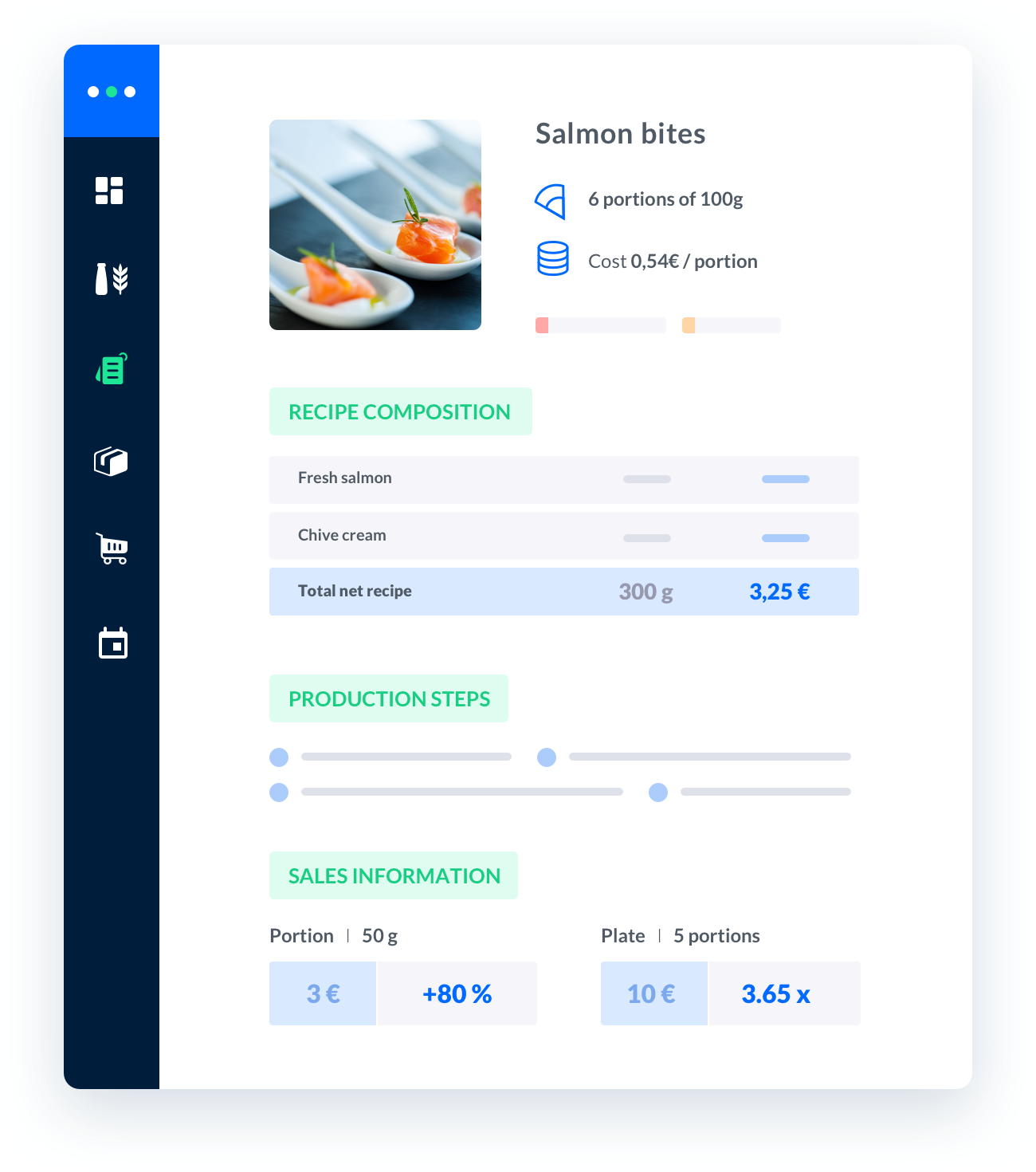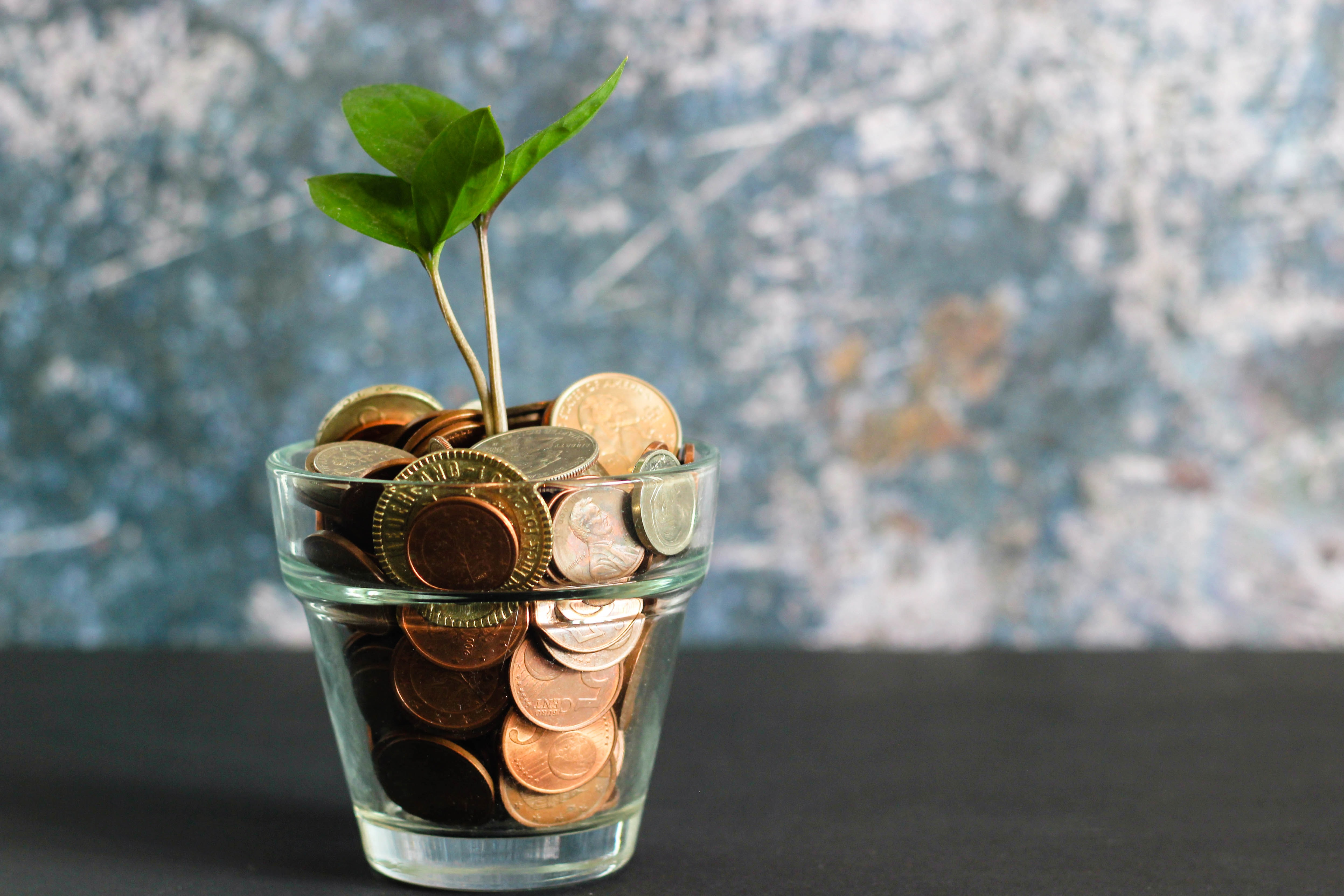# food costing formulas

Food Costing Formulas.Accurately calculating food costs is essential for any catering business.

Published on 01/01/2023Sébastien Vassaux##### Melba: the food cost app to optimize the profitability of your restaurant

Discover how to optimize the profitability of your restaurant with melba## Food Costing Formulas

Accurately calculating food costs is essential for any catering business. Here are some formulas to help you determine the cost of your food items:

## Ingredients Cost

The cost of ingredients is the most basic formula for calculating food costs. To calculate the cost of ingredients, simply add up the cost of each ingredient used in the dish.

Once you have calculated the cost of ingredients, you can use the following formula to calculate the cost of a menu item:

• Menu Item Cost = Cost of Ingredients + Labor Cost + Overhead Cost

## Food Cost Percentage

The food cost percentage is a measure of how much of your total revenue is spent on food. To calculate the food cost percentage, use the following formula:

• Food Cost Percentage = (Cost of Ingredients + Labor Cost + Overhead Cost) / Total Revenue

By using these formulas, you can accurately calculate the cost of your food items and ensure that your catering business is profitable.

## Take back control over your kitchen

Subscribe to our disrupting service to boost your productivity and profitability